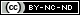## Maximum Value

29 08 2010

Sometimes you may wish to use PHP to do something as simple as finding the maximum numeric value in an array. There are two ways you can do that with a single dimension array. You could sort such an array, using sort() in the default ascending order and then select the last array element, as follows:

```
php
\$a = array(99,78,2,3,59,4,5);

sort(\$a);
echo 'max value is ' . \$a[count(\$a)-1];
/* or you could replace the last code line with: */
echo 'max value is ' . array_pop(\$a);
```

This algorithmic approach will correctly find that 99 is the largest number.

You could however simplify the code and get the correct result without even using sort(), as follows:

```<?php
\$a = array(99,78,2,3,59,4,5);
echo '<p>max value is ' . max(\$a);
```

This last approach hides the logic behind solving the problem. However, it is fast and requires less code. So, which approach is better? Probably this last one. Whenever you have a choice between creating code or using a built-in function, the built-in will usually be speedier since the built-ins are predominantly written in C and are already compiled.

Now let’s consider something more complex. How would you find the maximum numeric value in a multi-dimensional array? The answer may depend on the structure of the array. Let’s consider an irregular multi-dimensional array such as the following:

`\$a = array(2, 1, 4, 5,array(1200,1,10,array(130,24,2) ),3,19);`

What we have is an array whose fifth element is an array which in turn has a fourth element consisting of an array. I suppose one might be tempted to flatten things out so that we have only one array upon which we can apply max()

```<?php
// nested multi-dim
\$a = array(2,1,4,5,array(2200,1,10,array(130,24,2)),3,19);
\$temp = null;
function getValues( \$elem ) {
\$GLOBALS['temp'][] = \$elem;
}
array_walk_recursive(\$a,"getValues");
echo '<br />' . max(\$temp);
```

This solution involves using PHP’s handy array_walk_recursive() which takes minimally two parameters, the array for traversal and a call-back function. I use it to essentially create a flat array that contains every numeric value in \$a. You have probably heard that global variables are evil and that it’s preferable to avoid using them. In this particular case, the only way to create the flat array \$temp, is with a global variable and in this case it is \$temp. I refer to it in the call-back function using the superglobals array \$GLOBALS which stores the global variable’s name and value. I read somewhere that this superglobals array is preferable to using the global keyword. For those still unconvinced about the wisdom of using global variables in a function, you may wish to read http://tjhunt.blogspot.com/2009/04/php-global-variables-are-not.html.

The following is a different kind of multi-dimensional array. Here is an array where every element respresents an array and each contained array may be of varying length.

```// multi dim
\$b = array(
array(2, 1, 4, 5),
array(1200,1,10),
array(130,24,2),
array(3,19)
);
\$temp = array();
foreach (\$b as \$key => \$a) {

\$temp[] = max(\$a);
}
echo max(\$temp);
```

The above code refers to an array of arrays, so we can iterate through each array element without any need of recursion or functions with global variables. I apply max() to each element and assign the result to \$temp. Lastly I display the largest number by passing \$temp to max().

This last example, uses as multi-dimensional array whose elements are all arrays with the same number of elements. The second element is an associative array whose key is ‘prize’. If we wanted to find out the largest prize then we’d need to get the value of the second element in each array, assigning each to a new array and apply max(). We can do this as follows:

```// multi dim assoc
\$b =  array(
array(2,array('prize'=>1),4, 5),
array(1200,array('prize'=>8),10,99),
array(130,array('prize'=>24),2,55),
array(3,array('prize'=>19),1,2)
);
\$temp = array();
foreach (\$b as \$dex => \$array) {

foreach (\$array as \$key => \$elem) {

\$temp[] = \$elem['prize'];

}
}
echo max(\$temp);
```

Of the three multi-dimensional examples above, I like best the code in the first example. It works without one needing to know in advance how many nested arrays there are. If you have any other ideas about how to find the maximum value in a multi-dimensional array, please leave your suggestion in a comment.

This work is licensed under a.

### Information

This site uses Akismet to reduce spam. Learn how your comment data is processed.